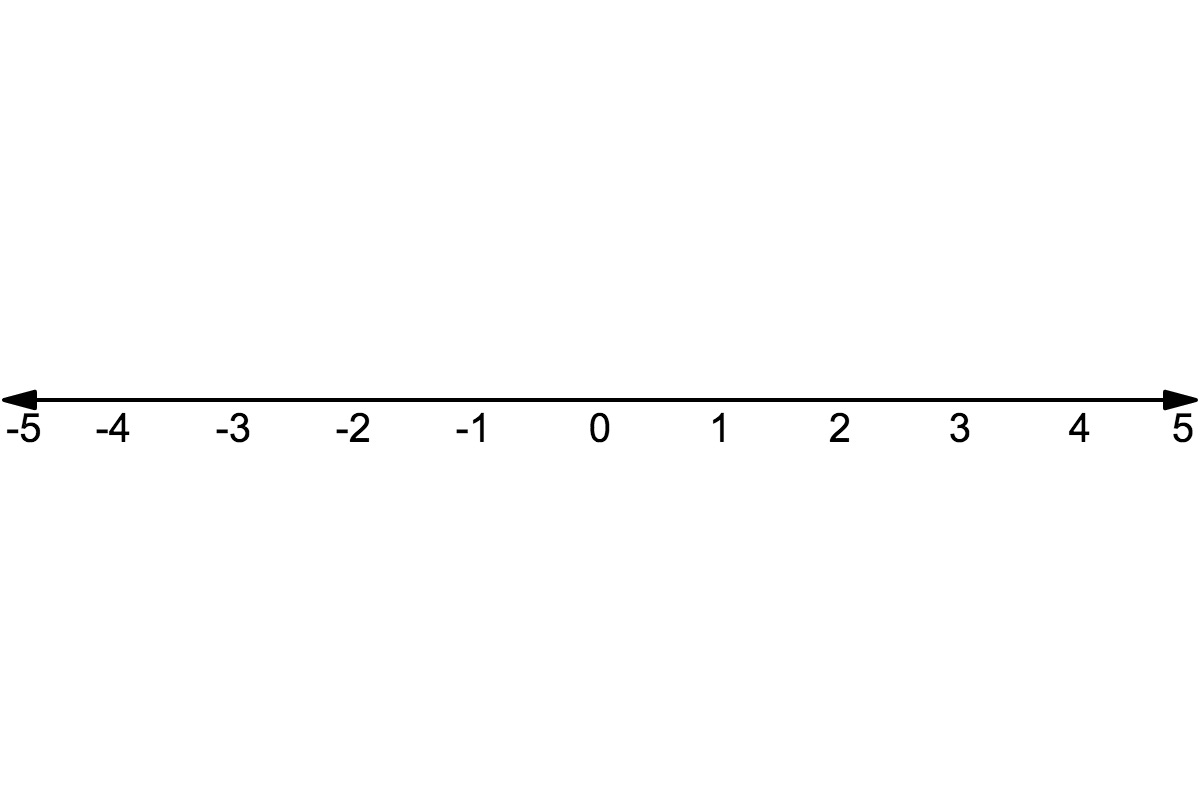# LINR 2 | Lesson 1 | Explore (Absolute Value)# Absolute Value

The absolute value of a number is the number’s distance away from zero.

Consider the values -2, 2, 1, -1, and 4, -4.    Place these numbers on the number-line.  What do you notice?   Can you come up with a generalization, for example given $$a$$ and $$-a$$?